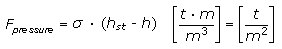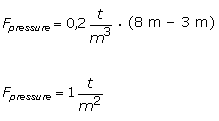1.3  Dynamic mechanical loads [German version]

Dynamic mechanical loads result from the movements of the means of transport and during handling. Dynamic loads are always given as a multiple of the acceleration of gravity. The acceleration of gravity is constant at 9.81 (∼10) m/s² = 1 g.

Dynamic loads cause packages to slip on loading areas, change the pressure loads on the lids, cause packaged goods to break free within the packages and distort the packages.

Static stacking loads are overlaid by dynamic components during transport and handling, which can result in the pressure load being increased or decreased. Vertical acceleration forces that occur during transport and handling are responsible for this. See Table 1.

If, for instance, a static load on the lid of 1 t/m² is overlaid by a dynamic vertical component of ± 0.8 g (see the Guidelines for Packing of Cargo Transport Units), this means that the load on the lid varies between 0.2 and 1.8 t/m².

For this reason, a load on the lid of 1.8 t/m² is always assumed when calculating the strength of boxes that will have packages stacked on them.

When loads are applied to the lids of boxes, care must be taken to ensure that such loads are distributed evenly. Suitable measures such as the use of wooden dunnage must be adopted to convert point loads and line loads to area loads.

If higher or lower stacking crush pressures are to be expected, the static stacking load is to be calculated using the following formula, where the weight of the load stacked above the package must be known:where:

 h = height of package [m] hst = stacking height [m] σ = mass (weight) per unit volume of the packages stacked above the current package [t/m³]

 Sample calculation: AssumptionsHeight of package = 3 mHeight of hold = 8 mMass per unit volume of the load stacked above the package = 0.2 t/m³In maritime transport, different hold heights result in higher stacking loads. This is less likely with land transport and when transporting containers.

The dynamic loads for the various means of transport are listed in various directives and standards:VDI 2700 For land transport by truckVDI 2700 / 7 For intermodal transportGuidelines for Packingof Cargo Transport Units For intermodal road-rail-sea transport operationsRID